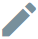# Application risk

With PassFort's Risk module, you can determine the risk that a profile's application poses to your company.

Contact us to find out how to get started with the Risk module.

### How risk is determined

When a profile starts a new product application, the risk model for that product is applied to the profile.

Every risk model includes two things:

• Risk factors: A risk factor is some detail about the profile that can influence the risk to your organisation (e.g. an individual's age, nationality, or PEPs matches). Each risk factor is assigned a score that shows how significant that factor is to the overall risk; the higher the score, the greater the risk. Any risk factor can be required or optional.
• Risk levels: A risk level indicates the overall risk the application poses to your company (e.g. Low risk, Medium risk, High risk). Each risk level has a range (e.g. 0-50), and if an application's total risk score falls within this range, the application will be assigned this risk level.

When a risk model is applied to an application, the risk factors are evaluated and a risk level is assigned.

An application's risk level can change throughout the course of onboarding and monitoring. The risk model is re-applied any time profile details used in the risk model change (e.g. the individual's birthday is reached, so the age changes).

Risk models are specific to profile type (i.e. individual or company). One product may have one risk model for individuals and another for companies.
When a risk model is re-applied to an application, all risk factors in the model are re-evaluated.

### See the risk of an application

The application's risk level is displayed:

• Next to its name in the Profiles list.
• Next to its name on the profile's Application overview page.
• Under Risk level on the profile's page for the application.
If the risk score could not be calculated, the risk level is Undetermined.
If the risk level is displayed as Calculating risk, the risk model is still being applied. Note that it may take some time to calculate the risk, especially in the case of company profiles that have many associates.

To learn more about how the risk level was determined, go to the profile's Application risk scores.

Every application that has a risk model is displayed. The risk level appears next to the application's name.

If two product applications are using the same risk model, each application will still be listed separately.

Click an application to see the breakdown of the risk level.

At the top of this breakdown, you'll see the overall risk score.

This is the cumulative score from every risk factor. It's used to determine which risk level is used for the application.

The risk level thresholds are displayed as well, so you can see exactly which threshold the overall risk score falls into and, therefore, which risk level is applied.

In the example above, the overall risk score is 50, which means the application falls into the 50-99 threshold of Medium risk.

Beneath that, you can see every risk factor in the risk model.

Risk factors can be combined into a group where the highest single risk score (Max of factors), lowest single risk score (Min of factors), mean risk score, or the sum of all risk scores is used. Click the name of a risk factor group to see which risk factors are included in it. In the example above, there is a risk factor group named Country risk factors, that takes the highest single score in the group.

If the risk factor must be evaluated before the risk score can be determined, it's marked Required. Otherwise, the risk score is optional and will only be used for the overall risk score if the relevant information is in the profile.

The Value shows what information the profile has for that risk factor. If the profile does not have information, the value is displayed as --. Risk factor groups always display --.

Action enables you to edit the profile information for that risk factor. To edit the information, click the Editbutton. If you cannot directly edit the information because the value for the factor is determined by the result of a check (e.g. PEPs matches), the action is displayed as --. Risk factor groups always display --.

The Score displays the risk score for that risk factor or risk factor group. This score is added to the application's overall risk score. If there is no value for the risk factor, the score is Undetermined.

It's possible for risk models to only have optional risk factors. If all the risk factors are optional and the profile does not have values for any of them, the overall risk score is 0.
If the risk factor is determined by running a check, you can go to the relevant task to run the check.

### Undetermined risk

If the risk level is Undetermined risk, the risk score could not be calculated because at least 1 required risk factor does not have a value.

To learn which risk factor(s) are missing, go to the profile's Application risk scores and click the application that has Undetermined risk.

For every risk factor marked Required:

1. Click the Editbutton. The Edit risk factor dialog is displayed.
2. Complete the information.
3. Click Update risk factor.

If the risk factor does not have an Editbutton, the value of the factor is determined by a check. Go to the relevant task and run the check.

Each time a value is added for a risk factor, the risk score is recalculated.